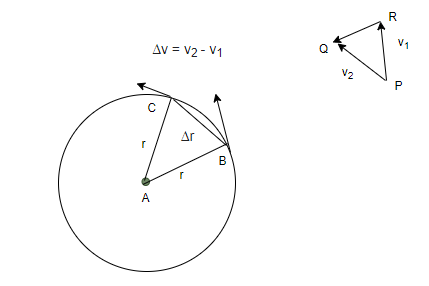GeeksforGeeks App
Open AppBrowser
Continue

# Centripetal Acceleration

There are a lot of objects around us in real life that are constantly performing the circular motion, even our planet revolves around the sun in a similar fashion. It is known that velocity is a vector quantity and any object performing circular motion is undergoing a change of velocity. Since there is a change in velocity, a force must be there that acts on the body to change its velocity continuously and make it perform the circular motion. It is essential to have an understanding of these forces to be able to understand the circular motion in detail. Let’s look at the concept of centripetal force and centripetal acceleration in detail.

### Centripetal Acceleration

Acceleration is defined as the rate of change of velocity. Now since acceleration and velocity both are vector quantities, they depend on direction. So, either change in the magnitude of the velocity and direction of the velocity. In a circular motion, there is always an acceleration because the velocity of the object is continuously changing. This acceleration is felt whenever a person is turning a car, there is a force acting on the person and the wheel too. The force depends on the radius of the turn and the velocity of the object. This means, the sharper the turn, the more force acts on the body.The figure given above shows an object which is moving in a circular motion. Assume that the speed of the object is constant. The figure shows the direction of the instantaneous velocity and points B and C. Since acceleration is the change in velocity, the change in velocity is roughly pointing towards the center of the curvature. To measure things instantaneously, the points B and C must be brought really closer and the angle between them should be infinitely small. In that, the change of velocity will point directly towards the center of the curvature.

Acceleration points towards the center of the curvature, now since the velocity is continuously changing and acceleration is present. This acceleration is called centripetal acceleration ac. Here, centripetal means “center seeking” or “toward the center”. Let’s derive the equation for calculating the magnitude of the centripetal force.

### Magnitude of Centripetal Acceleration

In the figure given above, notice that the triangles ABC and QPR are isosceles triangles. The two sides of the vector velocity triangles are denoted by v1 and v2. Comparing the two triangles using properties of similar triangles,The acceleration is given by the change in velocity with respect to time,Dividing both sides with delta-tRearranging the equation,Note that Δv/Δt is the ac and Δs/Δt is the tangential speed (v).

So, the centripetal acceleration becomes,

a = v2/r

This gives us the acceleration of an object under the circular motion traveling at a speed “v” and with radius “r”. This equation depends on the square of the velocity and inversely to the radius “r”.

Let’s look at some problems related to these concepts.

### Sample Problems

Question 1: Find the centripetal acceleration on an object performing circular motion with a radius of 5m. The velocity of the object is 10m/s.

The centripetal acceleration is given by,

a = v2/r

Given:

v = 10m/s.

r = 5m.

Plugging the values in the equation,

a = v2/r

⇒ a = (10)2/(5)

⇒ a = 100/5

⇒ a = 20 m/s2

Question 2: Find the centripetal acceleration on an object performing circular motion with a radius of 20m. The velocity of the object is 100m/s.

The centripetal acceleration is given by,

a = v2/r

Given:

v = 100m/s.

r = 20m.

Plugging the values in the equation,

a = v2/r

⇒ a = (100)2/(20)

⇒ a = 10000/20

⇒ a = 500 m/s2

Question 3: An object(m = 5Kg) is performing circular motion with radius 2m. If the velocity of the object is 8 m/s, find the centripetal force acting on the object.

The centripetal acceleration is given by,

a = v2/r

Given:

v = 8m/s.

r = 2m.

Plugging the values in the equation,

a = v2/r

⇒ a = (8)2/(2)

⇒ a = 64/2

⇒ a = 32 m/s2

Force acting on the object is given by,

F = ma

⇒ F = (5)(32)

⇒ F = 160 N

Question 4: An object(m = 2Kg) is performing circular motion with radius 5m. If the velocity of the object is 10 m/s, find the centripetal force acting on the object.

The centripetal acceleration is given by,

a = v2/r

Given:

v = 10m/s.

r = 5m.

Plugging the values in the equation,

a = v2/r

⇒ a = (10)2/(5)

⇒ a = 100/5

⇒ a = 20 m/s2

Force acting on the object is given by,

F = ma

⇒ F = (2)(20)

⇒ F = 40 N

Question 5: An object(m = 2Kg) is performing circular motion with radius 5m. If the centripetal force acting on the object is 100N, find the velocity of the object.

Force acting on the object is given by,

F = ma

⇒ 100 = (2)(a)

⇒ a = 50 m/s2

The acceleration of the object is given by,

a = v2/r

⇒ 50 = v2/5

⇒ 250 = v2

⇒ v = 5√10 m/s.

My Personal Notes arrow_drop_up
Related Tutorials